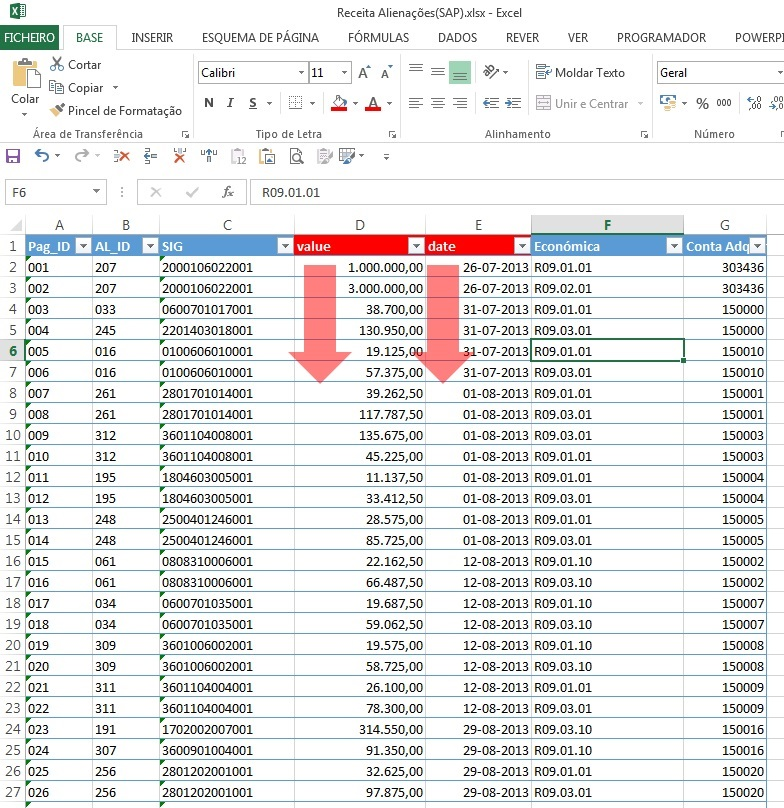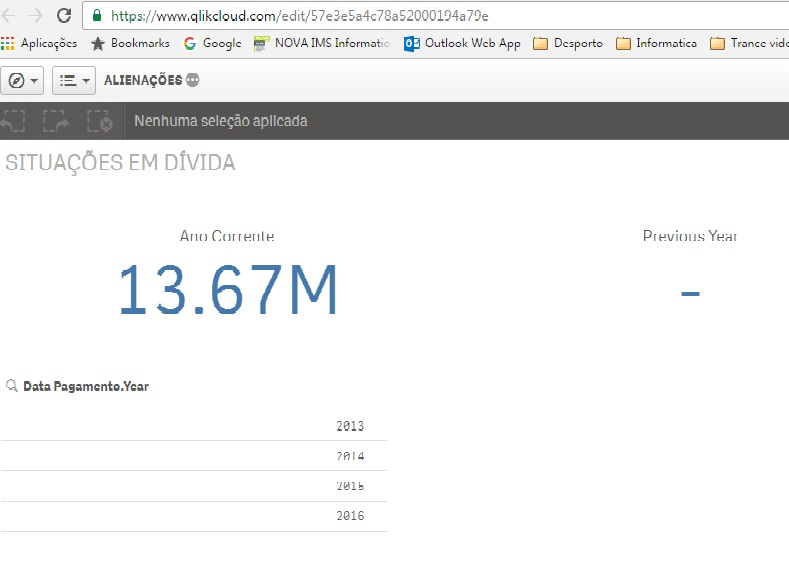# New to Qlik Sense

Discussion board where members can get started with Qlik Sense.

Announcements
Qlik® Product Spotlight: Discover what’s possible. Get more from our products.
See for yourself. Register today.Not applicable

## KPI Returns value from current and previous YearSomeone can give me a formula for previous year sum value, as shown in the pictures?Current Year:

Sum({\$<[Date] = {">=\$(=Yearstart(Max([Date])))  <= \$(=Date(Max([Date])))"}>} value)

Previous Year: ?

2 Replies
Valued Contributor

## Re: KPI Returns value from current and previous Year

Try with: Sum({\$<[Date] = {">=\$(=Yearstart(Max([Date]),-1))  <= \$(=YearEnd(Max([Date]),-1))"}>} value)

EduardoMVP

## Re: KPI Returns value from current and previous Year

Or this:

Sum({\$<[Date] = {">=\$(=YearStart(Max([Date]), -1))<=\$(=YearStart(Max([Date])) -1))"}>} value)

Community Browser